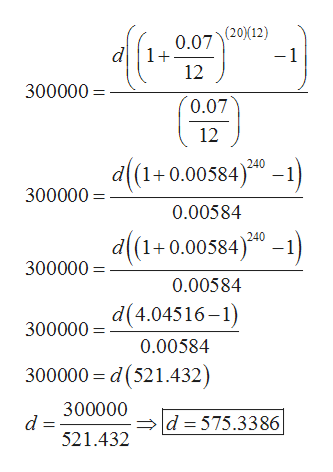# Suppose you want to have \$300,000 for retirement in 20 years. Your account earns 7% interest.a) How much would you need to deposit in the account each month?\$b) How much interest will you earn?\$

Question

Suppose you want to have \$300,000 for retirement in 20 years. Your account earns 7% interest.

a) How much would you need to deposit in the account each month?

\$

b) How much interest will you earn?

\$

check_circleExpert Solution
Step 1

According to the given information:

Amount earned after retirement PN=\$300,000

Time (n) = 20years

Rate of interest (r) = 7%

The amount to be deposited in the account each month can be calculated using the formula:

Step 2

Now put the values in th...help_outlineImage Transcriptionclose(20(12) -1 0.07 d1+ 12 300000 0.07 12 d(1+0.00584)0-) 240 300000 0.00584 d(1+0.005840- 300000 0.00584 d(4.04516-1) 300000 0.00584 300000 d(521.432) 300000 d 521.432 d 575.3386 fullscreen

### Want to see the full answer?

See Solution

#### Want to see this answer and more?

Solutions are written by subject experts who are available 24/7. Questions are typically answered within 1 hour*

See Solution
*Response times may vary by subject and question
Tagged in

### Math